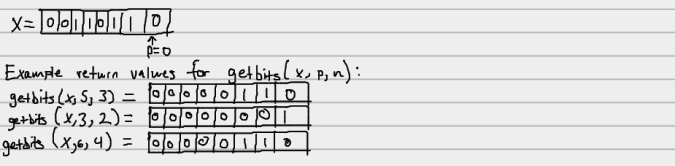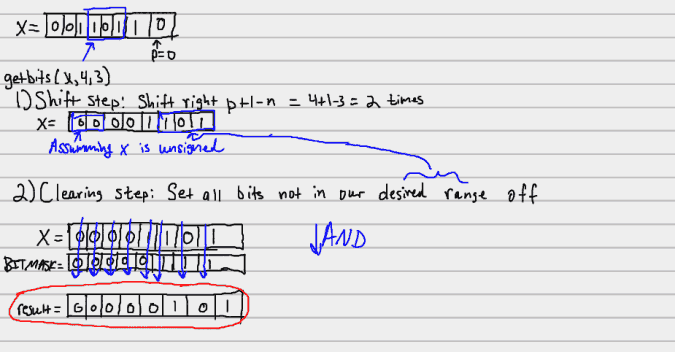## getbits() function

Suppose we have an integral value, and we would like to extract a certain number of bits at a particular position. Specifically, suppose we want to extract n bits from an integral starting at position p, where the rightmost bit is position 0.

For example:So how do we actually achieve the desired result? It takes two simple steps:

1. Shift our integral to the right until the last bit we are interested in sits at position 0.
2. Set all bits outside of our range of interest to 0

In the example above, we can see that getbits(x,5,3) took 3 shifts to the right to get the last bit we were interested in (the 0 at position 3) to position 0. It took getbits(x,3,2) 2 shifts, and getbits(x,6,4) 3 shifts. Thus the number of shifts needed can be generalized to p+1-n, and you can use the examples above to verify that claim.

Why we do need to set bits outside of our range of interest to 0? Because there may be bits set to 1 to the left of our p. We only want the n bits starting from p to be returned, so we must set all bits to the left of our range after the shift to 0.The way to generate this bitmask is to:

1. Start off with a sequence of all 1s
2. Shift to the left n times. This will place 0s in the vacant slots
3. Take the complement of the sequence, which will leave us with all 0s followed by 1s in the last n bits.

The getbits() function can thus be defined as so:

unsigned getbits(unsigned x, int p, int n) {
return (x >> (p+1-n)) & ~(~0 << n);
}


## (This post is part of the learning c series.)

Date published - December 03, 2013